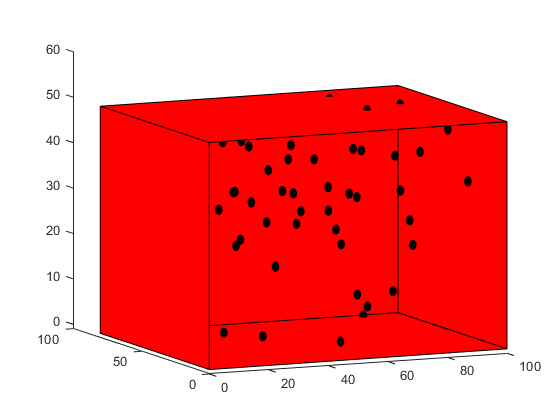2021-07-06 13:07

# MATLAB 三维长方体中的随机分布

• 写回答
• 关注问题
• 收藏
• 邀请回答

#### 1条回答默认 最新

•CSDN专家-Matlab_Fans 2021-07-06 22:17
已采纳

代码：

``````x = 100;
y = 80;
z = 50;
theta = linspace(0,2*pi,50);
phi = linspace(0,2*pi,50);
[theta,phi] = meshgrid(theta,phi);
r = 1;
X0 = r*cos(phi).*cos(theta);
Y0 = r*cos(phi).*sin(theta);
Z0 = r*sin(phi);

% 绘图
X = [0 x x 0
0 x x 0
0 0 0 0
x x x x
0 x x 0]';
Y = [0 0 y y
0 0 y y
0 y y 0
0 y y 0
y y y y]';
Z = [0 0 0 0
z z z z
0 0 z z
0 0 z z
0 0 z z ]';
figure
patch(X,Y,Z,'r');
view(3)
hold on
for ii = 1:50
p = [rand*100 rand*80 rand*50];    % 球心
X2= p(1) + X0;
Y2 = p(2) + Y0;
Z2 = p(3) + Z0;
patch( X2,Y2,Z2,'g' )
end
``````

圆半径0.1太小了，看不出效果，我改成1效果如下：打赏 评论